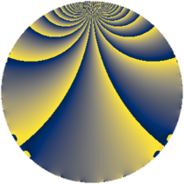# Properties

 Label 637.2.uLevel $637$ Weight $2$ Character orbit 637.u Rep. character $\chi_{637}(30,\cdot)$ Character field $\Q(\zeta_{6})$ Dimension $86$ Newform subspaces $10$ Sturm bound $130$ Trace bound $5$

# Related objects

## Defining parameters

 Level: $$N$$ $$=$$ $$637 = 7^{2} \cdot 13$$ Weight: $$k$$ $$=$$ $$2$$ Character orbit: $$[\chi]$$ $$=$$ 637.u (of order $$6$$ and degree $$2$$) Character conductor: $$\operatorname{cond}(\chi)$$ $$=$$ $$91$$ Character field: $$\Q(\zeta_{6})$$ Newform subspaces: $$10$$ Sturm bound: $$130$$ Trace bound: $$5$$ Distinguishing $$T_p$$: $$2$$, $$3$$

## Dimensions

The following table gives the dimensions of various subspaces of $$M_{2}(637, [\chi])$$.

Total New Old
Modular forms 146 102 44
Cusp forms 114 86 28
Eisenstein series 32 16 16

## Trace form

 $$86 q + 3 q^{2} - 4 q^{3} + 39 q^{4} + 6 q^{6} + 82 q^{9} + O(q^{10})$$ $$86 q + 3 q^{2} - 4 q^{3} + 39 q^{4} + 6 q^{6} + 82 q^{9} + 18 q^{10} + 2 q^{12} + 4 q^{13} - 15 q^{15} - 29 q^{16} - 11 q^{17} - 51 q^{18} + 6 q^{20} - 5 q^{23} + 35 q^{25} + 12 q^{26} - 22 q^{27} - 2 q^{29} - 52 q^{30} + 15 q^{31} - 9 q^{32} + 37 q^{36} + 27 q^{37} - 16 q^{38} + 27 q^{39} + 4 q^{40} + 15 q^{41} - 22 q^{43} + 48 q^{44} + 3 q^{45} + 42 q^{46} - 14 q^{48} + 30 q^{50} - 36 q^{51} - 52 q^{52} + 17 q^{53} + 9 q^{54} + 24 q^{55} - 21 q^{59} - 129 q^{60} - 4 q^{61} - 38 q^{62} - 16 q^{64} - 22 q^{65} + 43 q^{66} + 5 q^{68} - 7 q^{69} - 75 q^{71} + 57 q^{73} + 3 q^{74} - 3 q^{75} + 42 q^{76} - 123 q^{78} + 6 q^{79} + 46 q^{81} - 8 q^{82} - 9 q^{85} - 114 q^{86} - 13 q^{87} - 58 q^{88} - 36 q^{89} + 12 q^{90} + 62 q^{92} + 54 q^{93} - 28 q^{94} + 35 q^{95} + 12 q^{96} + 12 q^{97} + O(q^{100})$$

## Decomposition of $$S_{2}^{\mathrm{new}}(637, [\chi])$$ into newform subspaces

Label Dim $A$ Field CM Traces $q$-expansion
$a_{2}$ $a_{3}$ $a_{5}$ $a_{7}$
637.2.u.a $2$ $5.086$ $$\Q(\sqrt{-3})$$ None $$-3$$ $$2$$ $$3$$ $$0$$ $$q+(-2+\zeta_{6})q^{2}+q^{3}+(1-\zeta_{6})q^{4}+\cdots$$
637.2.u.b $2$ $5.086$ $$\Q(\sqrt{-3})$$ None $$3$$ $$-4$$ $$-3$$ $$0$$ $$q+(2-\zeta_{6})q^{2}-2q^{3}+(1-\zeta_{6})q^{4}+(-1+\cdots)q^{5}+\cdots$$
637.2.u.c $2$ $5.086$ $$\Q(\sqrt{-3})$$ None $$3$$ $$4$$ $$3$$ $$0$$ $$q+(2-\zeta_{6})q^{2}+2q^{3}+(1-\zeta_{6})q^{4}+(1+\cdots)q^{5}+\cdots$$
637.2.u.d $4$ $5.086$ $$\Q(\sqrt{-3}, \sqrt{-7})$$ None $$-3$$ $$-2$$ $$-3$$ $$0$$ $$q+(-1+\beta _{3})q^{2}+(-\beta _{1}-\beta _{3})q^{3}+(\beta _{1}+\cdots)q^{4}+\cdots$$
637.2.u.e $4$ $5.086$ $$\Q(\sqrt{-3}, \sqrt{-7})$$ None $$-3$$ $$2$$ $$3$$ $$0$$ $$q+(-1+\beta _{3})q^{2}+(\beta _{1}+\beta _{3})q^{3}+(\beta _{1}+\cdots)q^{4}+\cdots$$
637.2.u.f $4$ $5.086$ $$\Q(\sqrt{-3}, \sqrt{-13})$$ None $$6$$ $$0$$ $$0$$ $$0$$ $$q+(2-\beta _{2})q^{2}+(1-\beta _{2})q^{4}+\beta _{1}q^{5}+\cdots$$
637.2.u.g $12$ $5.086$ 12.0.$$\cdots$$.1 None $$0$$ $$-6$$ $$-3$$ $$0$$ $$q-\beta _{10}q^{2}+(-1+\beta _{1}-\beta _{3}-\beta _{8})q^{3}+\cdots$$
637.2.u.h $12$ $5.086$ 12.0.$$\cdots$$.1 None $$0$$ $$0$$ $$-6$$ $$0$$ $$q+\beta _{8}q^{2}+(-\beta _{2}-\beta _{4})q^{3}+(1-\beta _{2}+\cdots)q^{4}+\cdots$$
637.2.u.i $12$ $5.086$ 12.0.$$\cdots$$.1 None $$0$$ $$0$$ $$6$$ $$0$$ $$q+\beta _{8}q^{2}+(-\beta _{2}+\beta _{4})q^{3}+(1+\beta _{2}+\cdots)q^{4}+\cdots$$
637.2.u.j $32$ $5.086$ None $$0$$ $$0$$ $$0$$ $$0$$

## Decomposition of $$S_{2}^{\mathrm{old}}(637, [\chi])$$ into lower level spaces

$$S_{2}^{\mathrm{old}}(637, [\chi]) \cong$$ $$S_{2}^{\mathrm{new}}(91, [\chi])$$$$^{\oplus 2}$$